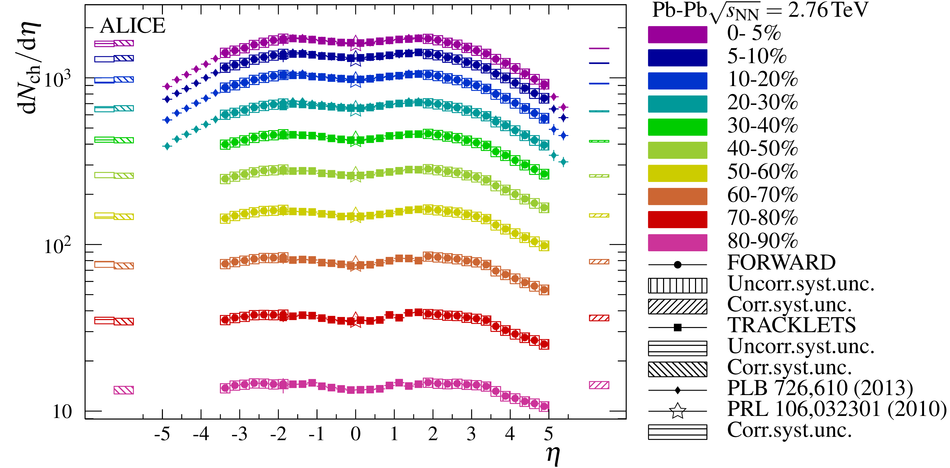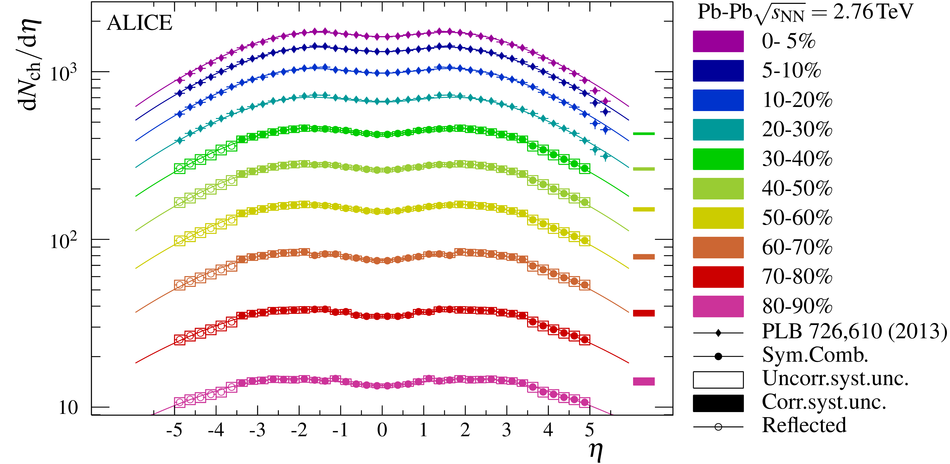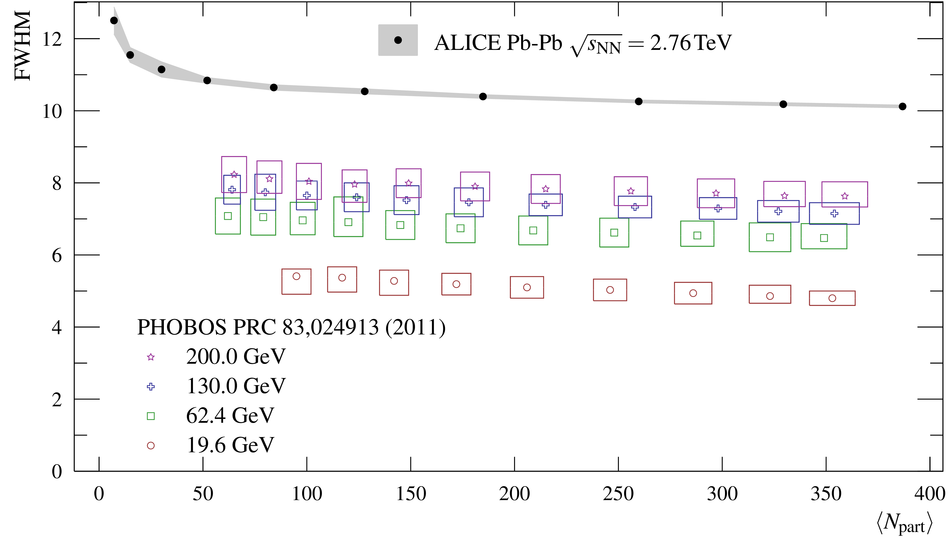# Centrality evolution of the charged-particle pseudorapidity density over a broad pseudorapidity range in Pb-Pb collisions at $\sqrt{s_{\rm NN}}$ = 2.76 TeV

The centrality dependence of the charged-particle pseudorapidity density measured with ALICE in Pb-Pb collisions at $\sqrt{s_{\rm NN}}$ over a broad pseudorapidity range is presented. This Letter extends the previous results reported by ALICE to more peripheral collisions. No strong change of the charged-particle pseudorapidity density distributions with centrality is observed, and when normalised to the number of participating nucleons in the collisions, the evolution over pseudorapidity with centrality is likewise small. The broad pseudorapidity range allows precise estimates of the total number of produced charged particles which we find to range from $162\pm22$ (syst.) to $17170\pm770$ (syst.) in 80-90% and 0-5 central collisions, respectively. The total charged-particle multiplicity is seen to approximately scale with the number of participating nucleons in the collision. This suggests that hard contributions to the charged-particle multiplicity are limited. The results are compared to models which describe $\mbox{d}N_{\mbox{ch}}/\mbox{d}\eta$ at mid-rapidity in the most central Pb-Pb collisions and it is found that these models do not capture all features of the distributions.

Figures

## Figure 1

 Comparison of data-driven to simulation-based corrections for secondary particles impinging on the FMD. Different markers correspond to different collision systems and energies, and the colours indicate the five FMD rings. $S(\eta)$ is shown for 0cm< IP$z$< 2cm as an example, while $E(\eta)$ is independent of IP$z$ (see also text). Pythia was used for pp collisions, and the Pb-Pb points are from simulation with a parameterisation which include the available ALICE data on particle composition and $\pT$ distributions. Black circles correspond to $E(\eta)$.## Figure 2

 Measurement of d$N_{ch}/$d$\eta$ per centrality from SPD (squares) and FMD (circles) separately. Error bars reflect the total uncorrelated systematic uncertainty and statistical error on each point. Error bars on the left and right reflect the correlated systematic uncertainties on the SPD and FMD points, respectively. Previously published results for 0-30% over the full pseudorapidity range (diamonds) and for 0-80% at mid-rapidity (stars)  are also shown.## Figure 3

 Measurement of d$N_{ch}/$d$\eta$ for all centralities and a broad $\eta$ range. Combined and symmetrised d$N_{ch}/$d$\eta$ over 30-90% centrality from both SPD and FMD (circles). Open boxes reflect the total uncorrelated systematic uncertainties and statistical errors, while the filled boxes on the right reflect the correlated systematic uncertainty. Also shown, is the reflection of the $3.5< \eta< 5$ values around $\eta=0$ (open circles). Previously published results for 0-30% over the full pseudorapidity range (diamonds)  are also shown. The lines correspond to fits of Eq.5 to the data.## Figure 4

 Full-width half-maximum of the charged-particle pseudorapidity distributions versus the average number of participants. The uncertainties on the ALICE measurements are from the fit of $f_{\rm{GG}}$ only and evaluated at $95\%$ confidence level. Also shown are lower energy results from PHOBOS .## Figure 5

 The charged--particle pseudorapidity density distributions scaled by the average number of participants in various pseudorapidity intervals as a function of the number of participants. The four right-most points (open symbols) in each $\eta$ range, as well as the mid-rapidity points (circles) are from previously published results [6,17]. The uncertainties on $\left\langle N_{\rm{part}}\right\rangle$ from the Glauber calculations are only included on the points at mid-rapidity. Thus, the uncertainty band around the mid-rapidity points reflect both the measurement uncertainties and the uncertainty on $\left\langle N_{\rm{part}}\right\rangle$, while other $\eta$ ranges only show the measurement uncertainties. The lower part shows the ratio of each distribution to the previously published distributions for $|\eta|< 0.5$.## Figure 6

 Extrapolation to the total number of charged particles as a function of the number of participating nucleons . The uncertainty on the extrapolation is smaller than the size of the markers. The four right-most points are the previously published results . A function inspired by factorisation  is fitted to the data, and the best fit yield $a=35.8\pm4.2$, $b=0.22\pm0.05$ with a reduced $\chi^2$ of $0.18$. Also shown is the PHOBOS result at lower energy result  scaled to the ALICE total number of charged particles per participant at $\langle N_{\text{part}}\rangle=180$.## Figure 7

 Comparison of d$N_{ch}/$d$\eta$ per centrality class from HIJING, AMPT (with and without string melting), and EPOS-LHC model calculations to the measured distributions.## Figure 1

 Ratio of the charged-particle pseudorapidity density distributions from $0$ to $80\%$ to the most peripheral distribution. Most systematic uncertainties in the forward region cancels, except for $2\%$ of the $6.1\%$ of the secondary correction $E(\eta)$ that stem from particle composition and $\pT$ distributions, nor does the additional $2\%$ in the FMD1 pseudorapidity acceptance cancel. At mid-rapidity, most systematic uncertainties do not cancel, except for the $0-2\%$ contribution from the acceptance correction, and about half of the contribution from extrapolating to $\pT=0$.## Figure 2

 Fit of $f_{T}$ of to the charged particle pseudorapidity density in 10 centrality bins.## Figure 3

 Fit of $f_{P}$ of to the charged particle pseudorapidity density in 10 centrality bins.## Figure 4

 Fit of $f_{P}$ of to the charged particle pseudorapidity density in 10 centrality bins.